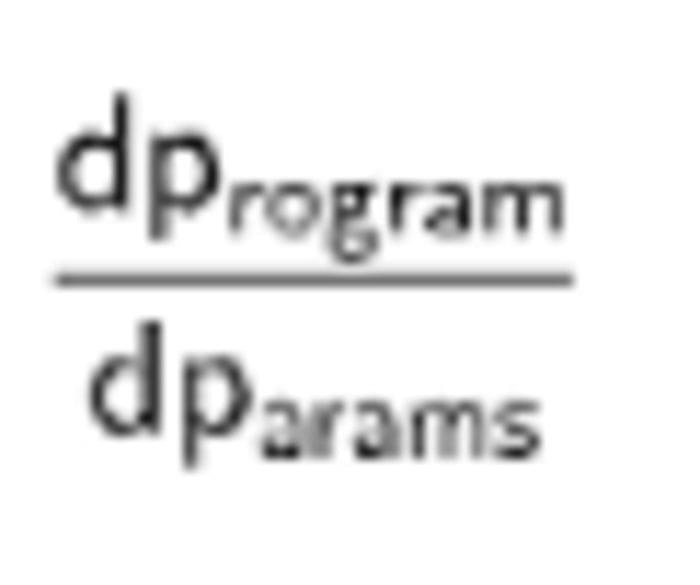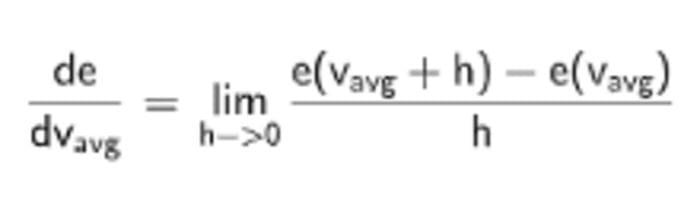# Differentiable Programming from Scratch

In this article, we are going to explain what Differentiable Programming is by developing from scratch all the tools needed for this exciting new kind of programming.

By Guillaume Saupin, CTO at Verteego

Last year I had the great opportunity to attend a talk with Yann Lecun, at the Facebook Artificial Intelligence Research Centre in Paris.

As a math enthusiast, what struck me during his talk is its rethinking of Deep Learning as Differentiable Programming. Yann Lecun has detailed his thoughts in a Facebook post:

An increasingly large number of people are defining the networks procedurally in a data-dependent way (with loops and conditionals), allowing them to change dynamically as a function of the input data fed to them. It’s really very much like a regular program, except it’s parameterized, automatically differentiated, and trainable/optimizable.

The overall goal of Differentiable Programming is to compute:Why do we need to be able to differentiate a program?

i.e to quantify the sensibility of the program and its outputs with respect to some of its parameters.

In this article, we are going to explain what Differentiable Programming is by developing from scratch all the tools needed for this exciting new kind of programming.

### The big picture

Let’s start with a simple toy example, showing how we’d write a program to estimate the duration of a taxi ride using traditional, data-independent code:

In this code, we compute the taxi trip duration using precomputed average speed in NYC: around 30km/h. This is the legacy way to make a program, i.e. data does not influence its parameters.

We use predefined parameters, here the average speed estimated by an expert, multiply the inverse of this speed by the trip distance, and we get the expected travel duration.

No matter how many times we run it, it will never improve. It will never learn from its error.

What Differentiable Programming offers is exactly the opposite: each run can be used to fine-tune the application parameters. Let’s see how this is accomplished.

### Leverage feedback

One thing that holds for computers as well as for humans is that to improve, you need feedback. And ideally, you need a way to quantify your mistakes.

In the computer world, this is done easily by introducing in our initial code a new function that computes a relatively common measurement of error: the squared error.

Code augmented with error computation

Once you get an idea of the error, you need a way to know in which direction you need to modify your parameters to reduce the error.

Let’s analyse a concrete example. Consider a trip whose duration was 12 minutes, and whose distance was 6km. To predict precisely this value with our model, the right parameter for the model would be 30 km/h. Any other value would result in an error for this trip.

Let’s have a look at the plot of squared error with respect to our param, the average speed, to get some insights. The whole code is straightforward:

The resulting curve is:The squared error is always positive, and more importantly, reaches its minimum for the right average speed. Image by Author.

The blue curve shows the evolution of trip duration with respect to speed. Faster trips obviously lead to smaller trip durations.

The orange curve displays the error as a simple difference between real duration, here 12 minutes, and the trip duration given the chosen speed. This error is zero for the real average speed: 30km/h.

The green curve is the squared error. Similarly to the error, zero is reached for an average speed of 30 km/h.

### Down the hill

We now have a way to quantify our error, with respect to the input parameter of our program. What we need now is some indication of how to reduce error.

Let’s say that our parameter, the average speed is 35km/h. The squared error is around 2.95. The question is, should we reduce speed or increase it to reduce the error? As we know the optimal value, 30 km/h, we obviously know that speed must be reduced. But for a real problem, we have to discover it.

Once again, let’s get insight from a graph that plots the squared error curve as well as the tangent to the curve for an average speed of 35 km/h.The error wrt to average speed, and the tangent at the curve for an average speed of 35km/h. Image by Author.

Looking at the tangent, it is clear that we need to follow its slope to decrease the error. In this case, this means that we should reduce the average speed. This method is called the gradient descent.

### Differentiation

The way to effectively know how to update our parameter is clear: we need to compute the tangent of our error function and estimate its slope. This means we must differentiate the error function to get its gradient.

There are various ways to compute the derivative of a function. For now, we will consider only two:

• Numerical differentiation, using the definition of differentiation based on limits
• Formal calculus, by derivation of the error formula with respect to average speed.

We’ll see a third, very versatile and robust option later in this article.

Here is the code used to plot the curve above:

The function speed_error_num_diff estimates error gradient with respect to error, using the derivative definition:Using the derivative definition allows for a simple and efficient calculation

As you can see, the code is straightforward but has the inconvenient of being numerically unstable and requiring an additional parameter: delta. Depending on the absolute value of the error, delta has to be tuned.

The alternative requires the symbolic derivation of the squared error formula:Formal differentiation of the error wrt average speed. Image by Author.

Both methods work and can be used to optimize the average speed parameter. We can plot iterations of the gradient descent method, and observe that the method converges as expected to 30 km/h:As we move the parameter in the gradient direction, the error is reduced. Image by Author.

The gradient descent is implemented with a loop and a constant, arbitrary coefficient that specifies the step size:

Code used for the optimizing the average speed parameter using gradient descent

### Automatic Differentiation

As shown above, these two methods works, but they are not always tractable. The formal one implies the symbolic derivation of the error formula, which is generally not as simple as in our example.

The numerical one is sometimes used but suffers from numerical instability due to round-off errors.

And both of them required the manual building of the gradient.

Fortunately, there is a method that automatically constructs all needed derivatives: Automatic Differentiation.

This method exploits two facts:

• In any program, we end up by making simple arithmetic operations (+-*/) and calling some fundamental functions like sin, cos, exp, log, …
• The chain rule can be used to differentiate complex mathematical expressions

To illustrate how this method works, we are going to develop a rudimentary implementation that supports only arithmetic operations. As a reminder, here is the list of derivation rules for the four arithmetic operations:Derivation rules for the (+-*/) operations. Image by Author.

There are two main implementations of Automatic Differentiation: the forward and reverse modes. As the forward mode is simpler to implement, we choose this one.

The principle is to augment number with an additional float, that stores the derivative. If the number is a constant, its derivative is zero, whereas if the number is a variable, its initial derivative with respect to itself is 1.0. This couple of floats is called a dual number and mathematically speaking constitutes an algebra on which we can perform standard operations.

All we have to do is create a class for DualFloat, that overloads the arithmetic operators, and uses the derivation rules above:

Now, let’s take the example of the polynomial f(x)=3x² and compute its derivative for x=4 using automatic differentiation manually.

3, being constant, is initialized with (3, 0), and x, the variable, is initialized with (4, 1). Using the newly defined calculation for dual number multiplication, we get:Calculation with dual numbers

And indeed, the derivative of f(x) is 6x, hence when x = 4f’(x)=24.

Using python, and the DualFloat class, we get:

We now have tools to rewrite the gradient descent using Automatic Differentiation:

Note that we don’t have to bother anymore with differentiating our calculus. All the necessary computations are done under the hood, using dual numbers. Calling derivative() on the error automatically provides the gradient.

### Differentiable Programming

We have developed in this article all the mathematical and programmatic tools to do Differentiable Programming. As you have seen, it is based on simple mathematical concepts and can be introduced in any program quite easily.

Note that is is not even necessary to rewrite code, as libraries exist that can be used as a substitute for numpy: see Jax for instance. The only remaining issue lies in the identification of the parameters that impact your program’s performances.

Once you have identified theses parameters and defined an error, you can compute the derivative of your parameters with respect to this error. Then each time you use new data, you can benefit from the free calculation of gradients to improve your parameters.

With Differentiable Programming, any program can benefit from all the mathematical tools available for differentiable functions, allowing them to improve themselves by learning from the data.

Bio: Guillaume Saupin is CTO at Verteego.

Original. Reposted with permission.

Related:

By subscribing you accept KDnuggets Privacy Policy

By subscribing you accept KDnuggets Privacy Policy

By subscribing you accept KDnuggets Privacy Policy